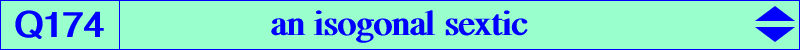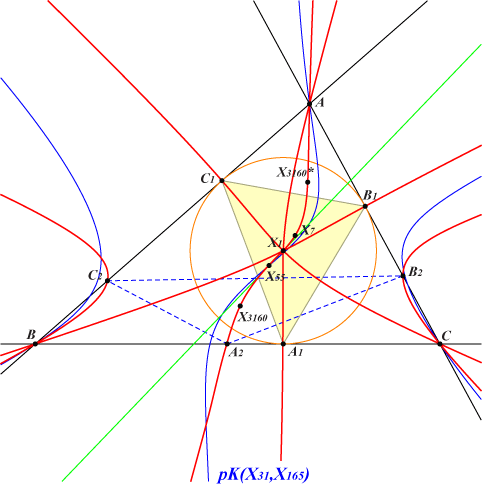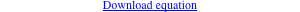too complicated to be written here. Click on the link to download a text file.X(1), X(7), X(55), X(3160) isogonal conjugate of X(3160) A1B1C1 : intouch triangle = cevian triangle of X(7) A2B2C2 : cevian triangle of X(3160)Q174 is analogous to Q172, where general properties are given. Let PaPbPc be the circumcevian triangle of a variable point P. With S = X(55), let X = BC ∩ SPa, Y = CA ∩ SPb, Z = AB ∩ SPc. ABC and XYZ are perspective (at Q) if and only if P lies on the cubic K1253 = pK(X31, X165), a circumcevian cubic of CL072. The locus of the perspector Q is the sextic Q174. Q174 is an isogonal sextic. X(1) is a quadruple point on Q174 and A, B, C are double points with tangents passing through X(55) and the isogonal conjugate of X(3160). The tangents at X(55), A1, B1, C1 pass through X(1). Q104 is another isogonal sextic with a very similar shape.